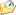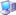* 帖子主题：MySQL 内置的坐标距离计算方法

st_distance(point(x1, y1), point(x2, y2))

st_distance_sphere(point(lon1, lat1), point(lon2, lat2))2022/11/24 13:34:22IP：已设置保密
pojin (ID: 2)

MySQL 还干这事？2022/11/24 13:51:43IP：已设置保密

Point X and Y coordinates are interpreted as longitude and latitude2022/11/24 13:55:05IP：已设置保密2022/11/24 13:56:32IP：已设置保密

PostgreSQL 也支持这个功能，但是，需要安装 postgis 扩展，此扩展比 postgresql 本身还大，共70个依赖（postgresql 才 19个），直接安装 postgis 时会自动安装 postgresql。

docker exec -ti -u postgres 容器ID psql
\c youdatabase
create extension postgis;

select st_distancesphere(point(0, 0)::geometry, point(1, 1)::geometry)

select *, pt2/pt1 as scalerate from (
select point_distance(point(116.420146, 39.897679), point(116.415122, 39.847263)) as pt1,
st_distancesphere(point(116.420146, 39.897679)::geometry, point(116.415122, 39.847263)::geometry) as pt2
) a2023/2/19 11:10:34IP：已设置保密

create or replace function distance_sphere
(in lon1 numeric, in lat1 numeric, in lon2 numeric, in lat2 numeric)
returns numeric
return asin(
sqrt(
sin(radians((lat1 - lat2) / 2)) ^ 2 +
sin(radians((lon1 - lon2) / 2)) ^ 2 *
)
) * 6371004 * 2

select distance_sphere(116.420146, 39.897679, 116.415122, 39.847263)2023/2/19 22:00:06IP：已设置保密

create or replace function distance_sphere
(in lon1 numeric, in lat1 numeric, in lon2 numeric, in lat2 numeric)
returns numeric return acos(
) * 63710042023/2/20 8:32:18IP：已设置保密
pojin (ID: 2)

======================================

select acos(2023/2/20 10:56:18IP：已设置保密